# Two cities

On a map, 1.5 cm represents 50 miles. If the distance between the two cities on the map is 6 cm, how many miles separate the cities?

x =  200 mi

### Step-by-step explanation:Did you find an error or inaccuracy? Feel free to write us. Thank you!Tips to related online calculators
Do you want to convert length units?

## Related math problems and questions:

• Scale of the map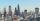The distance between two cities is actually 30 km and the map is 6 cm. What is the scale of the map?
• On the mapA line 1.5 cm long corresponds to a line 3 cm long on the map. What is the scale of the map?
• Nautical miles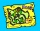How many nautical miles do they sail if the route is shown on a 1:25 000 scale map with a 7.4 cm long line?
• Map scaleGarden has on plan on a scale of 1: 150 width 22 cm and length 35 cm. What is the real area of the garden?
• Map 2At what scale is made map if the distance 8.2 km corresponds on the map segment 5 cm long?
• A map 4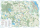A map represents every 4 miles with 1 inch. If a school and a bank are actually 12 miles apart, how far apart are they on the map, in inches?
• The scaleThe distance of 15 cm on the map corresponds to a distance of 27 km in reality. What is the scale of the map?
• Two monuments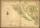The distance between two historical monuments on a map with a scale of 1: 500000 is 48 mm. Find the actual distance between these monuments in km.
• Map scale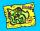What is the scale of the map, if 5.4 km is 72 mm on the map?
• A map 3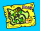A map is drawn to a scale of 1 : 25 000. This scale can be expressed as 1 cm represents n km. Find n.
• Scale of the mapDetermine the scale of the map if the actual distance between A and B is 720 km and distance on the map is 20 cm.
• The distance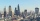The distance between two towns on a given map is 3 ½ cm. If ½ cm represents 6km. , what is the distance between the two towns?
• The sidewalk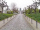The sidewalk is 50 m long. What will be its length on the plot plan in the scale of 1: 200?
• Map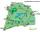On the tourist map at a scale of 1 : 50000 is distance between two points along a straight road 3.7 cm. How long travels this distance on a bike at 30 km/h? Time express in minutes.
• Two villages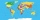Two villages are 11 km and 500 m away. On the map, their distance is determined by a 5 cm long line. Find the scale of the map.
• Scale of the mapDetermine the map's scale, which is the actual distance of 120 km l represented by a segment long 6 cm.
• The plan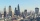The plan of the housing estate is in three scales 1: 5000,1: 10000,1: 15000. The distance between two points on a plan with a scale of 1: 10000 is 12 cm. What is this distance on the other two plans? What is this distance?